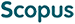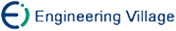侯 健, 彭家寅, 张宇卓, 张诚一. 基于极大模糊熵原理的模糊推理反向三I算法[J]. 计算机研究与发展, 2006, 43(7): 1180-1185.
 引用本文: 侯 健, 彭家寅, 张宇卓, 张诚一. 基于极大模糊熵原理的模糊推理反向三I算法[J]. 计算机研究与发展, 2006, 43(7): 1180-1185.Hou Jian, Peng Jiayin, Zhang Yuzhuo, Zhang Chengyi. A Reverse Triple I Algorithm for Fuzzy Reasoning Based on Maximum Fuzzy Entropy Principle[J]. Journal of Computer Research and Development, 2006, 43(7): 1180-1185.
 Citation: Hou Jian, Peng Jiayin, Zhang Yuzhuo, Zhang Chengyi. A Reverse Triple I Algorithm for Fuzzy Reasoning Based on Maximum Fuzzy Entropy Principle[J]. Journal of Computer Research and Development, 2006, 43(7): 1180-1185.## A Reverse Triple I Algorithm for Fuzzy Reasoning Based on Maximum Fuzzy Entropy Principle

• 摘要: 提出了用模糊熵来度量反向三I模糊推理结果的模糊程度，给出了模糊熵反向三I原则，讨论了FMP和FMT问题的模糊熵反向三I支持算法解存在的条件，分别给出了几个常见蕴涵算子的FMP问题与FMT问题的模糊熵反向三I解的计算公式.

Abstract: The fuzzy degree of a fuzzy reverse triple I inference conclusion is measured by fuzzy entropy, and a fuzzy entropy reverse triple I principle is introduced. Existence conditions of solutions are discussed for the fuzzy entropy reverse triple I algorithms for FMP and FMT problems, and the computing formulas of the algorithm based on some common implication operators are provided./下载:  全尺寸图片 幻灯片
• 分享
• 用微信扫码二维码

分享至好友和朋友圈